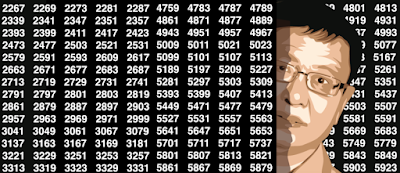## Sunday, April 1, 2018

### I Analyzed 10 MM digits of SQRT(2) - Look at My Findings

This article is intended for practitioners who might not necessarily be statisticians or statistically-savvy. The mathematical level is kept as simple as possible, yet I present an original, simple approach to test for randomness, with an interesting application to illustrate the methodology. This material is not something usually discussed in textbooks or classrooms (even for statistical students), offering a fresh perspective, and out-of-the-box tools that are useful in many contexts, as an addition or alternative to traditional tests that are widely used. This article is written as a tutorial, but it also features an interesting research result in the last section. The example used in this tutorial shows how intuiting can be wrong, and why you need data science.
The main question that we want to answer is: Are some events occurring randomly, or is there a mechanism making the events not occurring randomly? What is the gap distribution between two successive events of the same type? In a time-continuous setting (Poisson process) the distribution in question is modeled by the exponential distribution. In the discrete case investigated here, the discrete Poisson process turns out to be a Markov chain, and we are dealing with geometric, rather than exponential distributions. Let us illustrate this with an example.
Example
The digits of the square root of two (SQRT(2)), are believed to be distributed as if they were occurring randomly. Each of the 10 digits 0, 1, ... , 9 appears with a frequency of 10% based on observations, and at any position in the decimal expansion of SQRT(2), on average the next digit does not seem to depend on the value of the previous digit (in short, its value is unpredictable.)  An event in this context is defined, for example, as a digit being equal to (say) 3. The next event is the first time when we find a subsequent digit also equal to 3. The gap (or time elapsed) between two occurrences of the same digit is the main metric that we are interested in, and it is denoted as G. If the digits were distributed just like random numbers, the distribution of the gap G between two occurrences of the same digit, would be geometric